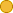## ← 01-46 Sense And Move

• 4 フォロワーs
• 31 Lines

dummy description

### 埋め込みコードを取得する x Embed video Use the following code to embed this video. See our usage guide for more details on embedding. Paste this in your document somewhere (closest to the closing body tag is preferable): ```<script type="text/javascript" src='https://amara.org/embedder-iframe'></script> ``` Paste this inside your HTML body, where you want to include the widget: ```<div class="amara-embed" data-url="http://www.youtube.com/watch?v=K8g3Hss8Q1A" data-team="udacity"></div> ``` 5言語

•英語(米国) [en] 原典
•スペイン語 [es] 未完了
•イタリア語 [it]
•日本語 [ja]
•ポルトガル語 [pt]

Showing Revision 4 created 05/30/2017 by Udacity Robot.

1. Wow, you've basically programmed the Google self-driving car localization
2. even though you might not quite know it yet.
3. Let me tell you where we are.
4. We talked about measurement updates, and we talked about motion.
5. We called these two routines "sense" and "move."
6. Now, localization is nothing else but the iteration of "sense" and "move."
7. There is an initial belief that is tossed into this loop if you.
8. If you sense first, if comes to the left side.
9. Then localization cycles through these--move, sense, move, sense, move, sense.
10. move, sense, move, sense cycle.
11. Every time the robot moves, it loses information as to where it is.
12. That's because robot motion is inaccurate.
13. Every time it senses it gains information.
14. That is manifest by the fact that after motion,
15. the probability distribution is a little bit flatter and a bit more spread out.
16. and after sensing, it's focused a little bit more.
17. In fact, as a foot note, there is a measure of information called "entropy."
18. Here is one of the many ways you can write it:
19. [-Ʃp(xi)log p(xi)]
20. as the expected log (logarithmic) likelihood of the probability of each grid cell.
21. Without going into detail, this is a measure of information that the distribution has,
22. and it can be shown that the update step, the motion step, makes the entropy go down,
23. and the measurement step makes it go up.
24. You really losing and gaining information.
25. I would now love to implement this in our code.
26. In addition to the 2 measurements we had before, red and green,
27. I'm going to give you 2 motions--1 and 1,
28. which means the robot moves right and right again.
29. Can you compute the posterior distribution if the robot first senses red,
30. then moves right by 1, then senses green, then moves right again?
31. Let's start with a uniform prior distribution.